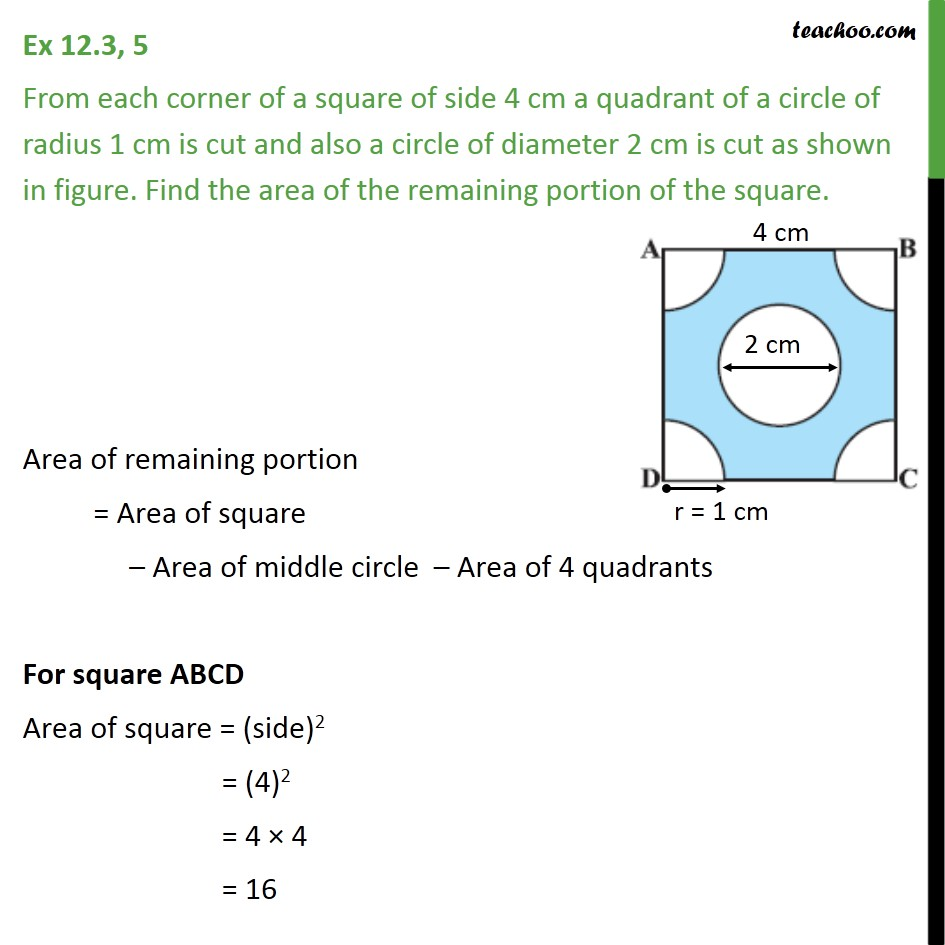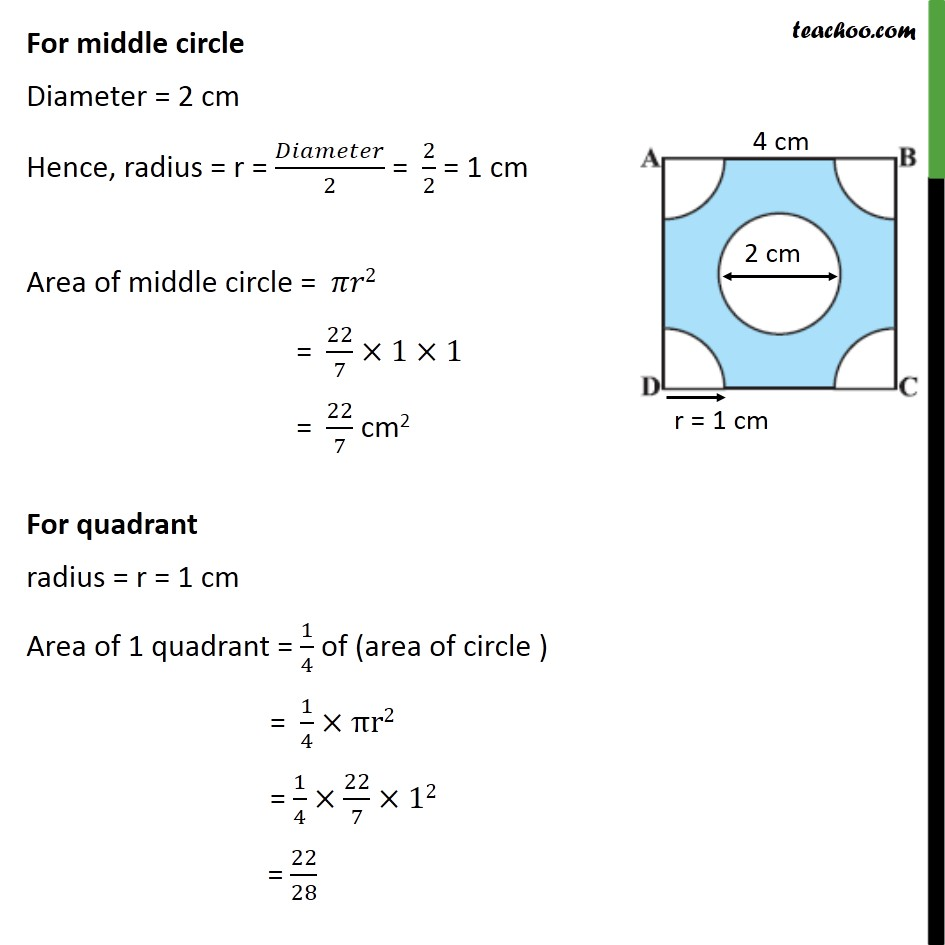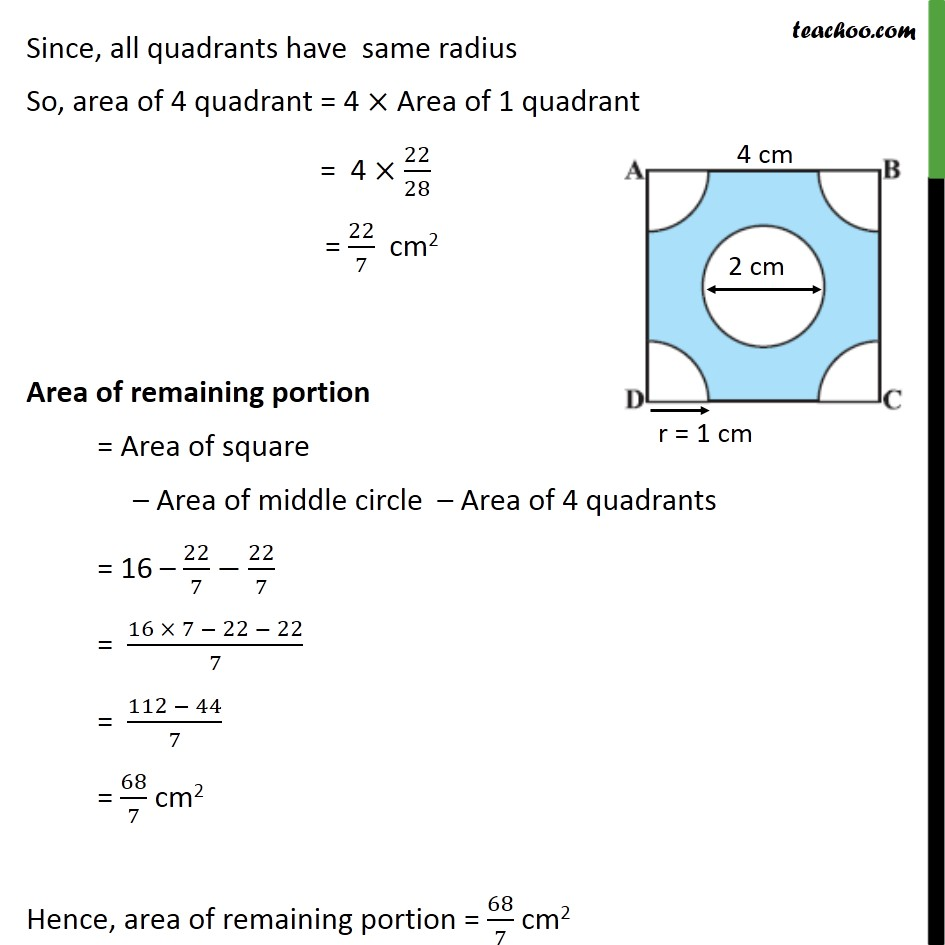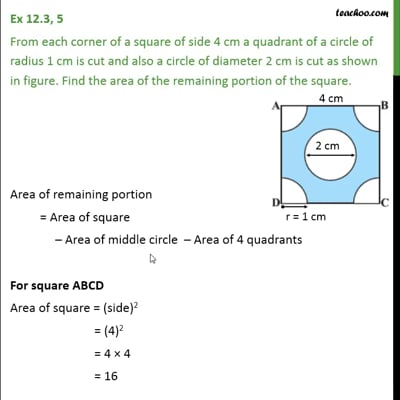Area of combination of figures : sector based

Chapter 12 Class 10 Areas related to Circles
Concept wiseThis video is only available for Teachoo black users

Solve all your doubts with Teachoo Black (new monthly pack available now!)

### Transcript

Ex 12.3, 5 From each corner of a square of side 4 cm a quadrant of a circle of radius 1 cm is cut and also a circle of diameter 2 cm is cut as shown in figure. Find the area of the remaining portion of the square. Area of remaining portion = Area of square – Area of middle circle – Area of 4 quadrants For square ABCD Area of square = (side)2 = (4)2 = 4 × 4 = 16 For middle circle Diameter = 2 cm Hence, radius = r = 𝐷𝑖𝑎𝑚𝑒𝑡𝑒𝑟/2 = 2/2 = 1 cm Area of middle circle = 𝜋𝑟2 = 22/7×1×1 = 22/7 cm2 For quadrant radius = r = 1 cm Area of 1 quadrant = 1/4 of (area of circle ) = 1/4×πr2 = 1/4×22/7×12 = 22/28 Since, all quadrants have same radius So, area of 4 quadrant = 4 × Area of 1 quadrant = 4 × 22/28 = 22/7 cm2 Area of remaining portion = Area of square – Area of middle circle – Area of 4 quadrants = 16 – 22/7−22/7 = (16 × 7 − 22 − 22)/7 = (112 − 44)/7 = 68/7 cm2 Hence, area of remaining portion = 68/7 cm2